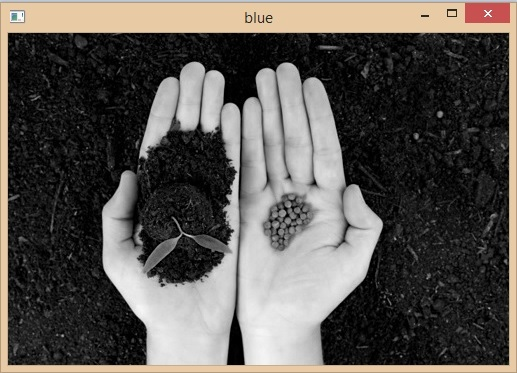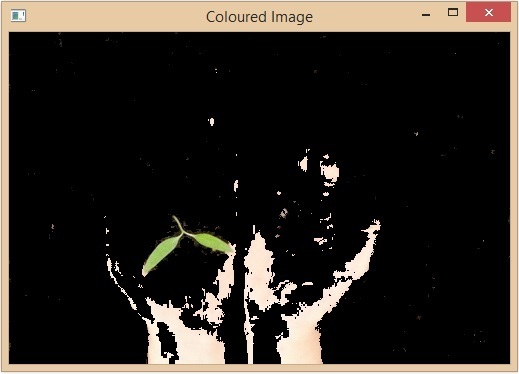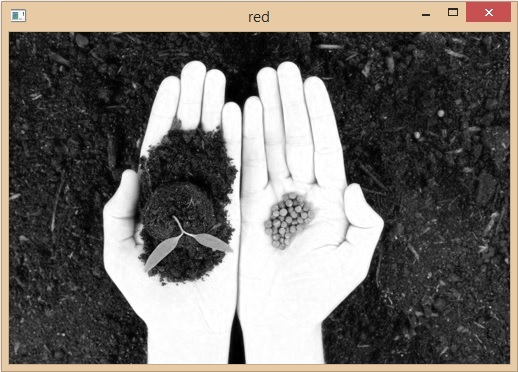# Color Spaces in OpenCV and Python

Color spaces are different types of color modes, used in image processing and signals and system for various purposes.

Open CV is a popular computer vision library written in C/C++ with bindings for Python, OpenCV provides easy ways of manipulating color spaces. Open CV is open source and gives various algorithms useful in image processing.

In computer vision, colors are represented using color spaces. There are so many color spaces that are currently used in computer vision and image processing, each having its own unique characteristics and advantages. Some of the very common color spaces in image processing are described below −

## RGB Color Space

Lets see about the RGB color space. It is one of the most commonly used and popular color spaces for image processing. In this color space the image is represented as a combination namaste of three colors Red, Green and Blue where each color has some value assigned to it this value ranges from 0 to 255. The more the value more is the presence of that color. By the combination of these 3 colors we can make all the possible colors in the visible spectrum.

The OpenCV cv2.imread() function can be used to read an image file and return a NumPy array representing the image's pixel values. The pixel values are represented as sets (B, G, R), where B, G and R represent the intensity values of the blue, green and red channels, respectively.

### Example

In the following example, we read an image and separate the different color channels of a particular image using the cv2.imshow() function.

This returns three separate arrays corresponding to the three colors Red, Green, and Blue by waiting in between for a key press between two images.

Then it displays these images with the corresponding colors. And closes them all at the end by destroyAllWindows() function at the last.

import cv2
B, G, R = cv2.split(image)

# Corresponding channels are separated
cv2.imshow("original", image)
cv2.waitKey(0)
cv2.imshow("blue", B)
cv2.waitKey(0)
cv2.imshow("Green", G)
cv2.waitKey(0)
cv2.imshow("red", R)
cv2.waitKey(0)
cv2.destroyAllWindows()


## Output

Original ImageBlue ImageGreen ImageRed Image## HSV Color Space

HSV stands for Hue, Saturation, and Value. The Hue channel represents the color's tint, while the Saturation channel represents the color's intensity or purity. The Value channel represents the brightness of the color.

It is particularly useful for color-based image segmentation tasks, where we need to isolate specific colors in an image. In OpenCV, we can convert an RGB image to the HSV color space using the cv2.cvtColor() function.

import cv2
import numpy as np

# Read an image in RGB color space

# show original image
cv2.imshow("original image",image)
cv2.waitKey(0)

# Convert the image to HSV color space
hsv_image = cv2.cvtColor(image, cv2.COLOR_BGR2HSV)

# show hsv image
cv2.imshow("hsv image",hsv_image)
cv2.waitKey(0)


## Output

Original Image −HSV Image −## YCbCr Color Space

The YCbCr color space separates the luminance (Y) and chrominance (CbCr) components of an image. The Y channel represents the brightness of the color, while the Cb and Cr channels represent the blue and red color differences, respectively.

It is particularly useful in video compression and transmission, where it can be used to reduce the amount of data required to represent an image or video stream.

In OpenCV, we can convert RGB image to YCbCr color space by passing cv2.COLOR_BGR2YCrCb and input image as parameter to cv2.cvtColor() function.

import cv2
import numpy as np

# Read an image in RGB color space

# show original image
cv2.imshow("original image",image)
cv2.waitKey(0)

# Convert the image to YCbCr color space
ycbcr_image = cv2.cvtColor(image, cv2.COLOR_BGR2YCrCb)

# show ycbcr image
cv2.imshow("ycbcr image", ycbcr_image)
cv2.waitKey(0)


## Output

Original Image −YCbCr Image −Some of the other color spaces are −

• LAB color space

• LUV color space

• XYZ color space

• CMYK color space

## Conclusion

In this article, we discussed some of the most commonly used color spaces in OpenCV and Python. We discussed about the RGB, HSV and the YCbCr color spaces. We alsolearnt how we can convert an image from one color space to another color space using the cv2.cvtColor() function from the OpenCV library. We understood about different color spaces and how each of them work in the OpenCV. We can use this to manipulate and analyze different images accordingly and extract the required information from them. We learnt about the advantages and disadvantages of different color space and what color space to choose for a specific task.

Updated on: 20-Apr-2023

1K+ Views